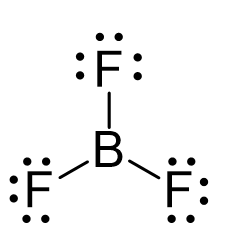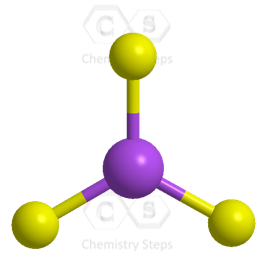## Examples

Boron is the central atom, so we can draw the skeletal structure as:There is a total of 3 + 3 x 7 = 24 electrons, and halogens on terminal positions are always going to have 3 lone pairs of electrons. Therefore, 3 x 6 = 18 electrons on the fluorenes, and the remaining 6 are used to make the three covalent bonds:The central atom has three bonds and no lone pairs, therefore, both the electron and molecular geometries are trigonal planar. The bond angle between the fluorine atoms is 120o.The steric number (the sum of the number of the atoms and lone pairs) of B is 3 which corresponds to sp2-hybridization.

Check Also